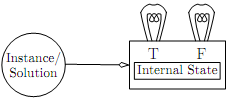## Abstract model for an algorithm solving a problem, Theory of Computation

Assignment Help:

These assumptions hold for addition, for instance. Every instance of addition has a unique solution. Each instance is a pair of numbers and the possible solutions include any third number. We can systematically list all instances along with all possible solutions by systematically listing all triples of numbers. This is not completely trivial-we can't, for instance, list all triples starting with 0 and then all triples starting with 1, etc. Since there are in?nitely many triples starting with zero, we would never get around to listing any starting with one. Suppose, though, that we are only concerned with the Natural Numbers, {0, 1, . . .}. If we ?rst list all triples that sum to zero (i.e., just the triple h0, 0, 0i) and then all triples that sum to one (i.e., h1, 0, 0i, h0, 1, 0i, h0, 0, 1i), etc., we are guaranteed that we will eventually list any given triple.

With the exception of the assumption that the solution is unique (which can be fudged in a variety of ways) these assumptions are pretty nearly minimal. We can't even consider solving a problem algorithmically unless every instance has a solution. An algorithm must produce some answer for every instance. If there is no answer for some instance, then whatever answer it produces will necessarily be wrong. (Note that if we modify the problem to require that we return "No Solution" in the case that none exists, we will have converted it into a problem that has a solution for every instance-albeit one that sometimes has the solution "No Solution".) The third assumption is true of every reasonable problem. In fact, it takes a fairamount of the theory of computation to even get to the point where we can argue that problems that don't satisfy the assumption might exist. Under these assumptions we can reduce our model to a machine for checking the correctness of solutions:#### Qbasic, Ask question #Minimum 100 words accepte

Ask question #Minimum 100 words accepte

#### NP complete, I want a proof for any NP complete problem

I want a proof for any NP complete problem

#### Applying the pumping lemma, Applying the pumping lemma is not fundamentally...

Applying the pumping lemma is not fundamentally di?erent than applying (general) su?x substitution closure or the non-counting property. The pumping lemma is a little more complica

#### Path function of a nfa, The path function δ : Q × Σ*→ P(Q) is the extension...

The path function δ : Q × Σ*→ P(Q) is the extension of δ to strings: Again, this just says that to ?nd the set of states reachable by a path labeled w from a state q in an

Rubber shortnote

#### Possibility of recognizing the palindrome language, Computer has a single F...

Computer has a single FIFO queue of ?xed precision unsigned integers with the length of the queue unbounded. You can use access methods similar to those in the third model. In this

#### Ardens theorem, how is it important

how is it important

#### Gastric juice, what are composition and its function of gastric juice

what are composition and its function of gastric juice

#### Exhaustive search, A problem is said to be unsolvable if no algorithm can s...

A problem is said to be unsolvable if no algorithm can solve it. The problem is said to be undecidable if it is a decision problem and no algorithm can decide it. It should be note

#### Finite automata, design an automata for strings having exactly four 1''s

design an automata for strings having exactly four 1''s